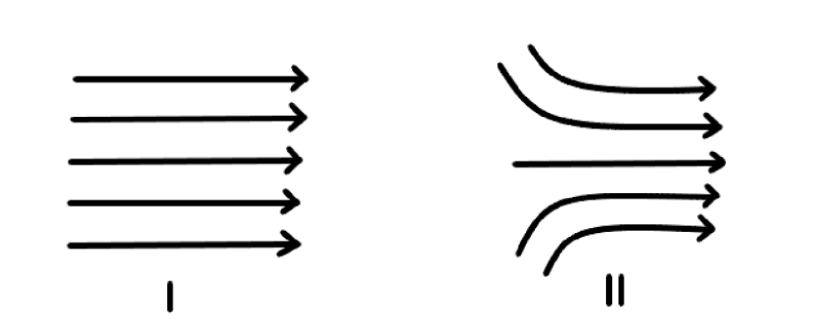QuestionAnswers

# Drawings $$I$$ and $II$ show two samples of electric field lines, then:A. The electric fields in both $I$ and $II$ are produced by negative charge located somewhere on the left and positive charges located somewhere on the right.B. In both $I$ and $II$ the electric field is the same everywhere.C. In both cases the field becomes stronger on moving from left to right.D. The electric field in $I$ is same everywhere, but in $II$ the electric field becomes strongeron moving from left to right.Verified
91.8k+ views
Hint:here, we will discuss each option one by one to find the correct option. As we know, the electric field lines emerge from a positive charge and go into a negative charge. Also, the density of the electric field lines will decide how stronger the electric field is.

As we know that the electric field lines always emerge from the positive charge and go into the negative charge. Therefore, in the above diagram, we can say that in both cases, the electric field lines are emerging from the positive charge and going into the negative.

Now, let us discuss each option given in the question.
In option (A), it is said that the electric field is produced by a negative charge and located on the left and the positive charge is located on the right. This option is wrong because electric field lines emerge from the positive charge, therefore, the positive charge will be on the left side and the negative charge will be on right.

Also, the electric field in the case $I$ are straight lines, therefore, the electric field will be the same in all directions. But in the case $II$ , the electric field lines are not straight, therefore, electric field lines are not the same everywhere in case $II$ . Therefore, option (B) is also incorrect.

Now, the density of electric field will be same in case $I$ , therefore, the electric field will same at all the points and in case $II$ , the density of electric field lines will be lower on the left side than on the right side, therefore, it will be stronger on the right side. Therefore, option (C) is also incorrect.

Therefore, the electric field will be the same in the case $I$, but in case $II$ , the electric field becomes stronger as we move from left to right.

Hence, option (D) is the correct option.

NOTE:Here, the density of electric field lines in the case $II$ is greater on the right side than the left side. This is because the electric field lines are concentrated more on the right side than the left side.

Therefore, the electric field will be stronger on the right side than the left side.